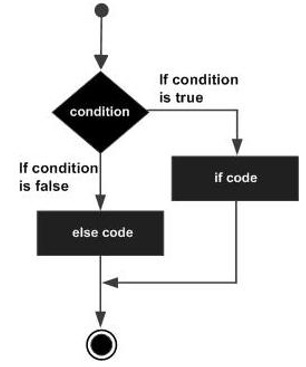# Groovy - If/Else Statement

The next decision-making statement we will see is the if/else statement. The general form of this statement is −

```if(condition) {
statement #1
statement #2
...
} else{
statement #3
statement #4
}
```

The general working of this statement is that first a condition is evaluated in the if statement. If the condition is true it then executes the statements thereafter and stops before the else condition and exits out of the loop. If the condition is false it then executes the statements in the else statement block and then exits the loop. The following diagram shows the flow of the if statement.Following is an example of a if/else statement −

```class Example {
static void main(String[] args) {
// Initializing a local variable
int a = 2

//Check for the boolean condition
if (a<100) {
//If the condition is true print the following statement
println("The value is less than 100");
} else {
//If the condition is false print the following statement
println("The value is greater than 100");
}
}
}
```

In the above example, we are first initializing a variable to a value of 2. We are then evaluating the value of the variable and then deciding on which println statement should be executed. The output of the above code would be

```The value is less than 100.
```
groovy_decision_making.htm